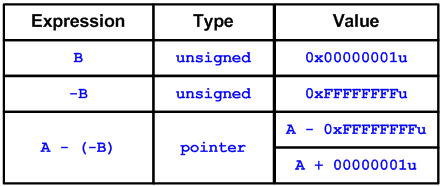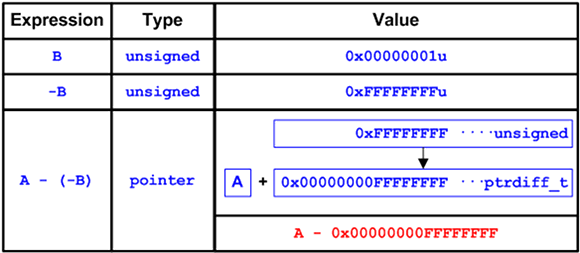﻿ Why A + B != A - (-B)# Why A + B != A - (-B)

While developing Viva64 analyzer intended for detecting 64-bit errors, I sometimes encounter interesting ways of code behavior. I would like to show you one example that is not very interesting practically but might be helpful in understanding more complicated cases.

``````char *A = "123456789";
unsigned B = 1;
char *X = A + B; // X: "23456789"
char *Y = A - (-B); // Y: <Bad Ptr>``````

If we compile the 32-bit version of the code, the expressions "A + B" and "A - (-B)" will be equivalent. In the 32-bit code, the pointers X and Y point to the second item of the array A. To understand it better look at the Figure 1 showing the process of calculating "A - (-B)".But when we compile the 64-bit code, the expressions "A + B" and "A - (-B)" mean absolutely different things. The subexpression "-B" has an unsigned type and equals 0xFFFFFFFFu. And it is this value 0xFFFFFFFFu that is subtracted from the pointer (see Figure 2).The shown error leads to an access outside the array on a 64-bit system. Such errors might occur when working with negative indexes when 32-bit unsigned variables are used to store them. Here is an example:

``````unsigned Index = -1;
Array[Index] = Z;``````

Like in the previous case, the expression "Array[Index] = Z;" works well in the 32-bit program but leads to an error in the 64-bit one.

Conclusion:

You should avoid using unsigned data types to store negative values. If the variables used to access array items can take negative values, use only signed data types, for example "int". Or rather use the types size_t and ptrdfiff_t.##### Use PVS-Studio to search for bugs in C, C++, C# and Java

We offer you to check your project code with PVS-Studio. Just one bug found in the project will show you the benefits of the static code analysis methodology better than a dozen of the articles.

412
14 132

### Do you make errors in the code?